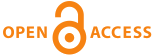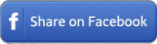Home|Journals|Articles by Year|Audio Abstracts RSS - TOCin any field in Title in Summary in Keywords in Authors in journalsEEO. 2020; 19(1): 472-475

##### ON THE NON-LINEAR DIOPHANTINE EQUATION 𝒑𝒙 + (𝒑 + 𝟐)𝒚 = 𝒛𝟐

*Sani Gupta, Rajiv Kumar, Satish Kumar.

##### Abstract

: In this paper, we consider the non-linear Diophantine equation
𝑝𝑥+ (𝑝 + 2)𝑦 = 𝑧2  (1)
Where p and p+2 are twin primes and x, y and z are positive integers with zero. We find that the equation (1) has infinite solutions (p, x, y, z) = ( 6n-1, 1, 1, 2 3𝑛 ) for some natural numbers n and other possible solution (3, 1, 0, 2,) of equation (1) also considered.

Key words: Diophantine Equations, Exponential Equations, Congruency Relation, Twin Prime

Full-text options

 Share this ArticleOnline Article Submission
• ejmanager.com